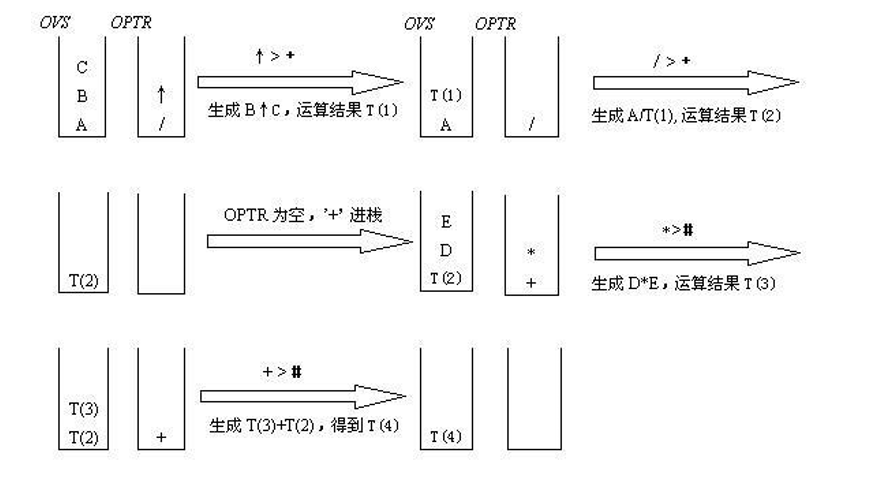（这题总让我想到一些不好的往事）。

``````void BracketMatch(char *str)
/* str[]中为输入的字符串，利用堆栈技术来检查该字符串中的括号是否匹配*/
{
Stack S; int i; char ch;
InitStack(&S);
For(i=0; str[i]!=’\0’; i++)   /*对字符串中的字符逐一扫描*/
{
switch(str[i]){
case ‘(‘:Push(&S,str[i]); break;
case ‘[‘:Push(&S,str[i]); break;
case ‘{‘:Push(&S,str[i]); break;
case ‘)’:
if(IsEmpty(&S))
{
printf("\n右括号多余!");
return;
}
else
{
GetTop (&S,&ch);
if(Match(ch,str[i]))  /*用Match判断两个括号是否匹配*/
Pop(&S,&ch);  /*已匹配的左括号出栈*/
else
{
printf("\n对应的左右括号不同类!");
return;
}
}
break;

case ‘]’:
if(IsEmpty(&S))
{
printf("\n右括号多余!");
return;
}
else
{
GetTop (&S,&ch);
if(Match(ch,str[i]))  /*用Match判断两个括号是否匹配*/
Pop(&S,&ch);  /*已匹配的左括号出栈*/
else
{
printf("\n对应的左右括号不同类!");
return;
}
}
break;
case ‘}’:
if(IsEmpty(&S))
{
printf("\n右括号多余!");
return;
}
else
{
GetTop (&S,&ch);
if(Match(ch,str[i]))  /*用Match判断两个括号是否匹配*/
Pop(&S,&ch);  /*已匹配的左括号出栈*/
else
{
printf("\n对应的左右括号不同类!");
return;
}
}  break;

if(IsEmpty(&S))
printf("\n括号匹配!");
else
printf("\n左括号多余!");
}
``````

（1）规定运算符的优先级表
（2）设置两个栈：OVS(运算数栈)、OPTR(运算符栈)
（3）自左向右扫描，进行如下处理：

1、如果当前运算符>OPTR 栈顶运算符，则当前运算符进 OPTR 栈；
2、如果当前运算符≤OPTR 栈顶运算符，则 OPTR 退栈一次，得到栈顶运算符θ，OVS 连续退栈两次，得到运算数 a、运算数 b，对 a，b 执行θ运算，得到结果 T(i)，将 T(i) 进OVS 栈。``````int ExpEvaluation()
/*读入一个简单算术表达式并计算其值。operator和operand分别为运算符栈和运算数栈，
OPS为运算符集合*/
{
InitStack(&operand);
InitStack(&operator);
Push(&operator,'#');
printf("\n\nPlease input an expression (Ending with #) :");  ch=getchar();
while(ch!='#'||GetTop(operator)!='#') /* GetTop()通过函数值返回栈顶元素*/
{
if(!In(ch,OPS))   /*不是操作符，是操作数*/
{
n=GetNumber(ch);push(&operand,n);
}
else
switch(Compare(ch, GetTop(operator))) //比较运算符的优先级
{
case '>':  Push(&operator,ch);   ch=getchar();  break;
case '=':
case '<':
Pop(&operator,&op);
Pop(&operand,&b);
Pop(&operand,&a);
v=Execute(a,op,b);  /* 对a和b进行op运算 */
Push(&operand,v); break;
}
}
v=GetTop(operand); return (v);
}
``````